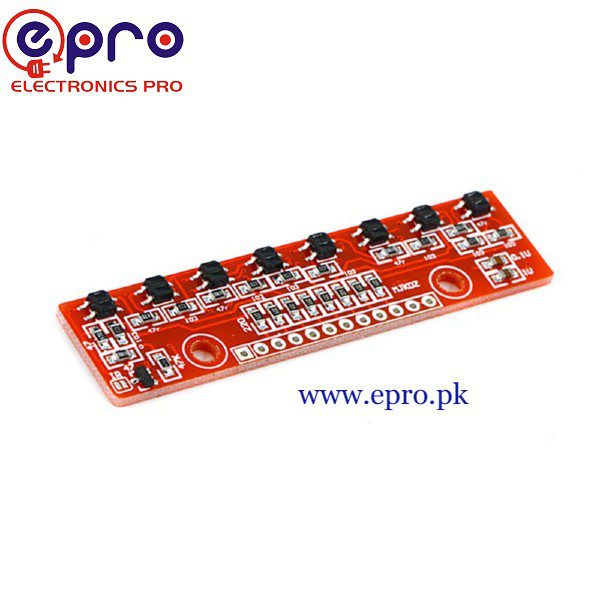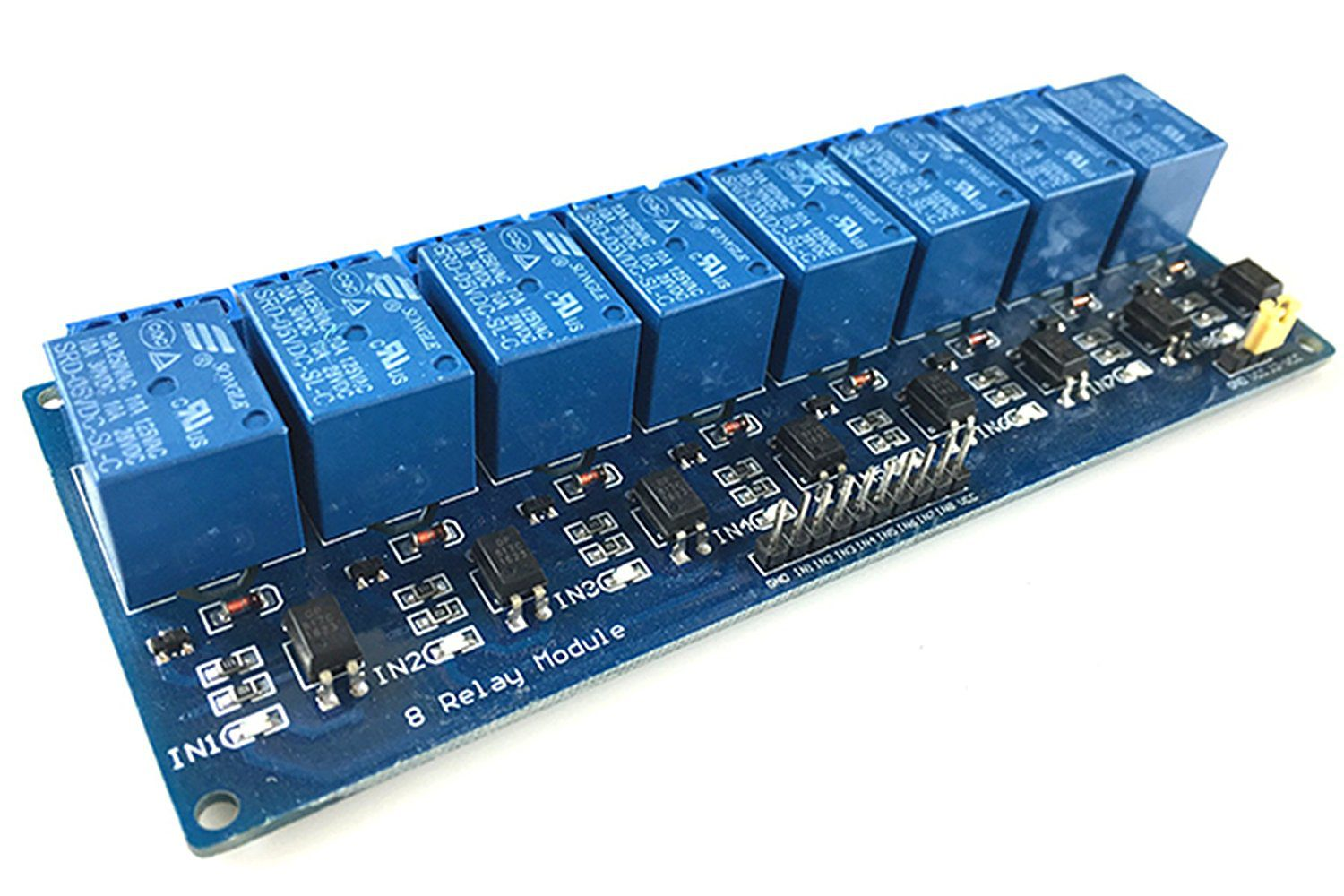# 8 Channel520.00

## QTR-8RC Line Following Sensor in Pakistan

0 out of 5
(0)
SKU: N/A

### Specifications:

• Its dimensions is 2.95 x 0.5 x 0.125 inches.
• Its operating voltages are 3.3 to 5 volts.
• Sensing distance is almost 3mm
• Its supply current amount is 100mA.
• It is digital Input-output pins compatible.
• Its optimal distance is 0.125 inches.
• Its maximum sensing distance is 0.375 inches.
• Its weight is 0.11 oz (without pins)## QTR-8RC Line Following Sensor in Pakistan

0 out of 5
(0)

### Specifications:

• Its dimensions is 2.95 x 0.5 x 0.125 inches.
• Its operating voltages are 3.3 to 5 volts.
• Sensing distance is almost 3mm
• Its supply current amount is 100mA.
• It is digital Input-output pins compatible.
• Its optimal distance is 0.125 inches.
• Its maximum sensing distance is 0.375 inches.
• Its weight is 0.11 oz (without pins)
SKU: N/A
520.00## QTR-8RC Line Following Sensor in Pakistan

0 out of 5
(0)

### Specifications:

• Its dimensions is 2.95 x 0.5 x 0.125 inches.
• Its operating voltages are 3.3 to 5 volts.
• Sensing distance is almost 3mm
• Its supply current amount is 100mA.
• It is digital Input-output pins compatible.
• Its optimal distance is 0.125 inches.
• Its maximum sensing distance is 0.375 inches.
• Its weight is 0.11 oz (without pins)
520.00## QTR-8RC Line Following Sensor in Pakistan

0 out of 5
(0)

### Specifications:

• Its dimensions is 2.95 x 0.5 x 0.125 inches.
• Its operating voltages are 3.3 to 5 volts.
• Sensing distance is almost 3mm
• Its supply current amount is 100mA.
• It is digital Input-output pins compatible.
• Its optimal distance is 0.125 inches.
• Its maximum sensing distance is 0.375 inches.
• Its weight is 0.11 oz (without pins)
520.00

Showing all 2 results